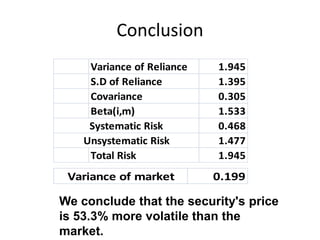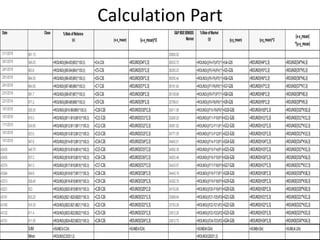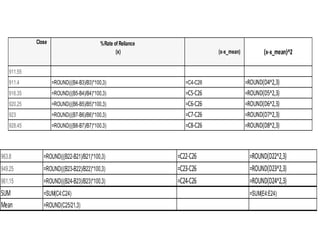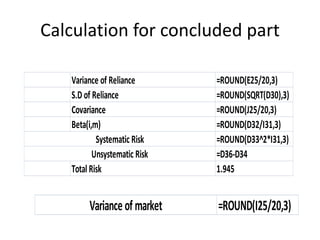Publicité

# SAPM Assignment on Reliance Industries.pptx

24 Mar 20231 sur 7
Publicité

### SAPM Assignment on Reliance Industries.pptx

1. SAPM Assignment on Reliance Industries. From- Alkesh Khare Student id- JLU02967
2. Values for checking the volatility of the Reliance company 1/1/2018 911.55 33812.75 2/1/2018 911.4 -0.016 -0.278 0.077 33812.26 -0.001 -0.296 0.088 0.082 3/1/2018 916.35 0.543 0.281 0.079 33793.38 -0.056 -0.351 0.123 -0.099 4/1/2018 920.25 0.426 0.164 0.027 33969.64 0.522 0.227 0.052 0.037 5/1/2018 923 0.299 0.037 0.001 34153.85 0.542 0.247 0.061 0.009 8/1/2018 928.45 0.59 0.328 0.108 34352.79 0.582 0.287 0.082 0.094 9/1/2018 940.9 1.341 1.079 1.164 34443.19 0.263 -0.032 0.001 -0.035 10/1/2018 941.5 0.064 -0.198 0.039 34433.07 -0.029 -0.324 0.105 0.064 11/1/2018 937.2 -0.457 -0.719 0.517 34503.49 0.205 -0.09 0.008 0.065 12/1/2018 946.75 1.019 0.757 0.573 34592.39 0.258 -0.037 0.001 -0.028 15/1/2018 947.6 0.09 -0.172 0.03 34843.51 0.726 0.431 0.186 -0.074 16/1/2018 923.5 -2.543 -2.805 7.868 34771.05 -0.208 -0.503 0.253 1.411 17/1/2018 924.65 0.125 -0.137 0.019 35081.82 0.894 0.599 0.359 -0.082 18/1/2018 919.3 -0.579 -0.841 0.707 35260.29 0.509 0.214 0.046 -0.18 19/1/2018 929.35 1.093 0.831 0.691 35511.58 0.713 0.418 0.175 0.347 22/1/2018 971.2 4.503 4.241 17.986 35798.01 0.807 0.512 0.262 2.171 23/1/2018 981.7 1.081 0.819 0.671 36139.98 0.955 0.66 0.436 0.541 24/1/2018 964.55 -1.747 -2.009 4.036 36161.64 0.06 -0.235 0.055 0.472 25/1/2018 964.55 0 -0.262 0.069 36050.44 -0.308 -0.603 0.364 0.158 29/1/2018 963.8 -0.078 -0.34 0.116 36283.25 0.646 0.351 0.123 -0.119 30/1/2018 949.25 -1.51 -1.772 3.14 36033.73 -0.688 -0.983 0.966 1.742 31/1/2018 961.15 1.254 0.992 0.984 35965.02 -0.191 -0.486 0.236 -0.482 SUM 5.498 38.902 6.201 3.982 6.094 Mean 0.262 0.295 Date %Rate of Reliance (x) (x-x_mean) Close S&P BSE SENSEX Market (x-x_mean)^2 (y-y_mean)^2 (x-x_mean) *(y-y_mean) %Rate of Market (y) (y-y_mean)
3. Conclusion We conclude that the security's price is 53.3% more volatile than the market. Variance of Reliance 1.945 S.D of Reliance 1.395 Covariance 0.305 Beta(i,m) 1.533 0.468 1.477 Total Risk 1.945 Systematic Risk Unsystematic Risk 0.199 Variance of market
4. Calculation Part 31/1/2018 961.15 35965.02 30/1/2018 949.25 =ROUND(((B4-B3)/B3)*100,3) =C4-C26 =ROUND(D4^2,3) 36033.73 =ROUND(((F4-F3)/F3)*100,3) =G4-G26 =ROUND(H4^2,3) =ROUND(D4*H4,3) 29/1/2018 963.8 =ROUND(((B5-B4)/B4)*100,3) =C5-C26 =ROUND(D5^2,3) 36283.25 =ROUND(((F5-F4)/F4)*100,3) =G5-G26 =ROUND(H5^2,3) =ROUND(D5*H5,3) 25/1/2018 964.55 =ROUND(((B6-B5)/B5)*100,3) =C6-C26 =ROUND(D6^2,3) 36050.44 =ROUND(((F6-F5)/F5)*100,3) =G6-G26 =ROUND(H6^2,3) =ROUND(D6*H6,3) 24/1/2018 964.55 =ROUND(((B7-B6)/B6)*100,3) =C7-C26 =ROUND(D7^2,3) 36161.64 =ROUND(((F7-F6)/F6)*100,3) =G7-G26 =ROUND(H7^2,3) =ROUND(D7*H7,3) 23/1/2018 981.7 =ROUND(((B8-B7)/B7)*100,3) =C8-C26 =ROUND(D8^2,3) 36139.98 =ROUND(((F8-F7)/F7)*100,3) =G8-G26 =ROUND(H8^2,3) =ROUND(D8*H8,3) 22/1/2018 971.2 =ROUND(((B9-B8)/B8)*100,3) =C9-C26 =ROUND(D9^2,3) 35798.01 =ROUND(((F9-F8)/F8)*100,3) =G9-G26 =ROUND(H9^2,3) =ROUND(D9*H9,3) 19/1/2018 929.35 =ROUND(((B10-B9)/B9)*100,3) =C10-C26 =ROUND(D10^2,3) 35511.58 =ROUND(((F10-F9)/F9)*100,3) =G10-G26 =ROUND(H10^2,3) =ROUND(D10*H10,3) 18/1/2018 919.3 =ROUND(((B11-B10)/B10)*100,3) =C11-C26 =ROUND(D11^2,3) 35260.29 =ROUND(((F11-F10)/F10)*100,3) =G11-G26 =ROUND(H11^2,3) =ROUND(D11*H11,3) 17/1/2018 924.65 =ROUND(((B12-B11)/B11)*100,3) =C12-C26 =ROUND(D12^2,3) 35081.82 =ROUND(((F12-F11)/F11)*100,3) =G12-G26 =ROUND(H12^2,3) =ROUND(D12*H12,3) 16/1/2018 923.5 =ROUND(((B13-B12)/B12)*100,3) =C13-C26 =ROUND(D13^2,3) 34771.05 =ROUND(((F13-F12)/F12)*100,3) =G13-G26 =ROUND(H13^2,3) =ROUND(D13*H13,3) 15/1/2018 947.6 =ROUND(((B14-B13)/B13)*100,3) =C14-C26 =ROUND(D14^2,3) 34843.51 =ROUND(((F14-F13)/F13)*100,3) =G14-G26 =ROUND(H14^2,3) =ROUND(D14*H14,3) 43435 946.75 =ROUND(((B15-B14)/B14)*100,3) =C15-C26 =ROUND(D15^2,3) 34592.39 =ROUND(((F15-F14)/F14)*100,3) =G15-G26 =ROUND(H15^2,3) =ROUND(D15*H15,3) 43405 937.2 =ROUND(((B16-B15)/B15)*100,3) =C16-C26 =ROUND(D16^2,3) 34503.49 =ROUND(((F16-F15)/F15)*100,3) =G16-G26 =ROUND(H16^2,3) =ROUND(D16*H16,3) 43374 941.5 =ROUND(((B17-B16)/B16)*100,3) =C17-C26 =ROUND(D17^2,3) 34433.07 =ROUND(((F17-F16)/F16)*100,3) =G17-G26 =ROUND(H17^2,3) =ROUND(D17*H17,3) 43344 940.9 =ROUND(((B18-B17)/B17)*100,3) =C18-C26 =ROUND(D18^2,3) 34443.19 =ROUND(((F18-F17)/F17)*100,3) =G18-G26 =ROUND(H18^2,3) =ROUND(D18*H18,3) 43313 928.45 =ROUND(((B19-B18)/B18)*100,3) =C19-C26 =ROUND(D19^2,3) 34352.79 =ROUND(((F19-F18)/F18)*100,3) =G19-G26 =ROUND(H19^2,3) =ROUND(D19*H19,3) 43221 923 =ROUND(((B20-B19)/B19)*100,3) =C20-C26 =ROUND(D20^2,3) 34153.85 =ROUND(((F20-F19)/F19)*100,3) =G20-G26 =ROUND(H20^2,3) =ROUND(D20*H20,3) 43191 920.25 =ROUND(((B21-B20)/B20)*100,3) =C21-C26 =ROUND(D21^2,3) 33969.64 =ROUND(((F21-F20)/F20)*100,3) =G21-G26 =ROUND(H21^2,3) =ROUND(D21*H21,3) 43160 916.35 =ROUND(((B22-B21)/B21)*100,3) =C22-C26 =ROUND(D22^2,3) 33793.38 =ROUND(((F22-F21)/F21)*100,3) =G22-G26 =ROUND(H22^2,3) =ROUND(D22*H22,3) 43132 911.4 =ROUND(((B23-B22)/B22)*100,3) =C23-C26 =ROUND(D23^2,3) 33812.26 =ROUND(((F23-F22)/F22)*100,3) =G23-G26 =ROUND(H23^2,3) =ROUND(D23*H23,3) 43101 911.55 =ROUND(((B24-B23)/B23)*100,3) =C24-C26 =ROUND(D24^2,3) 33812.75 =ROUND(((F24-F23)/F23)*100,3) =G24-G26 =ROUND(H24^2,3) =ROUND(D24*H24,3) SUM =SUM(C4:C24) =SUM(E4:E24) =SUM(G4:G24) =SUM(I4:I24) =SUM(J4:J24) Mean =ROUND(C25/21,3) =ROUND(G25/21,3) Date %RateofReliance (x) (x-x_mean) Close S&PBSESENSEX Market (x-x_mean)^2 (y-y_mean)^2 (x-x_mean) *(y-y_mean) %RateofMarket (y) (y-y_mean)
5. 911.55 911.4 =ROUND(((B4-B3)/B3)*100,3) =C4-C26 =ROUND(D4^2,3) 916.35 =ROUND(((B5-B4)/B4)*100,3) =C5-C26 =ROUND(D5^2,3) 920.25 =ROUND(((B6-B5)/B5)*100,3) =C6-C26 =ROUND(D6^2,3) 923 =ROUND(((B7-B6)/B6)*100,3) =C7-C26 =ROUND(D7^2,3) 928.45 =ROUND(((B8-B7)/B7)*100,3) =C8-C26 =ROUND(D8^2,3) %Rate of Reliance (x) (x-x_mean) Close (x-x_mean)^2 963.8 =ROUND(((B22-B21)/B21)*100,3) =C22-C26 =ROUND(D22^2,3) 949.25 =ROUND(((B23-B22)/B22)*100,3) =C23-C26 =ROUND(D23^2,3) 961.15 =ROUND(((B24-B23)/B23)*100,3) =C24-C26 =ROUND(D24^2,3) SUM =SUM(C4:C24) =SUM(E4:E24) Mean =ROUND(C25/21,3)
6. Calculation for concluded part =ROUND(I25/20,3) Variance of market Variance of Reliance =ROUND(E25/20,3) S.D of Reliance =ROUND(SQRT(D30),3) Covariance =ROUND(J25/20,3) Beta(i,m) =ROUND(D32/I31,3) =ROUND(D33^2*I31,3) =D36-D34 Total Risk 1.945 Systematic Risk Unsystematic Risk
7. THANK YOU
Publicité